``library(tidyverse)``
``## ── Attaching packages ─────────────────────────────────────────────────────────────────────────────────────────────────────────────────────── tidyverse 1.3.0 ──``
``````## ✓ ggplot2 3.3.2     ✓ purrr   0.3.4
## ✓ tibble  3.0.3     ✓ dplyr   1.0.2
## ✓ tidyr   1.1.1     ✓ stringr 1.4.0
## ✓ readr   1.3.1     ✓ forcats 0.5.0``````
``````## ── Conflicts ────────────────────────────────────────────────────────────────────────────────────────────────────────────────────────── tidyverse_conflicts() ──

## Introduction

Complex systems in our everyday lives are almost always made up of several steps or operations that must be performed sequentially. Some examples are manufacturing processes like building an electronic product, which comprises gathering raw materials, then going through a sequential process of steps involving separating and modifying raw materials, to develop an intermediate product, which later goes through another series of sequential steps before the final product is manufactured. In the medical field, an example of such a complex system of processes is drug testing, what with the current COVID-19 pandemic the world is facing. In the aviation industry, production of jet engine components is one such complex system, wherein a series of steps are performed to produce a jet engine component from raw materials.

Given that we are concerned with applications of machine learning in the real world, this project aims to provide insights into such potential real-world applications, through training a regression model, using several methods, then using these models to predict a final binary response.

## Overview

In this project, we are provided with `11` continuous inputs `x01` to `x11`, `2` categorical inputs `xA` and `xB`, and `1` intermediate response, `response_1`. Our primary aim is to optimize the complex system, and identify the important input variables that minimize the probability that the component will fail the inspection — these inputs would then represent the best input settings to use on the machines in each step of the manufacturing process.

This project will delve into two main approaches to building a binary classifier to predict if the component will fail the inspection:

1. considering all `11` continuous and `2` categorical inputs as the inputs to the binary classifier, and
2. considering `response_1` as an input along with the `5` continuous inputs `x07` to `x11`, and `2` categorical inputs.

This investigation is divided into `5` main parts:

1. Exploration
2. Regression models
3. Binary classification option b
4. Binary classification option a
5. Interpretation and optimization

This first R Markdown file tackles part i, specifically visualizing the data.

## Final project data

The code chunk below reads in the data for this project.

``````data_url <- 'https://raw.githubusercontent.com/jyurko/CS_1675_Fall_2020/master/HW/final_project/cs_1675_final_project_data.csv'

``````## Parsed with column specification:
## cols(
##   xA = col_character(),
##   xB = col_character(),
##   x01 = col_double(),
##   x02 = col_double(),
##   x03 = col_double(),
##   x04 = col_double(),
##   x05 = col_double(),
##   x06 = col_double(),
##   x07 = col_double(),
##   x08 = col_double(),
##   x09 = col_double(),
##   x10 = col_double(),
##   x11 = col_double(),
##   response_1 = col_double(),
##   outcome_2 = col_character()
## )``````

Get a glimpse of the data.

``df %>% glimpse()``
``````## Rows: 2,013
## Columns: 15
## \$ xA         <chr> "A1", "A1", "A1", "A1", "A1", "A1", "A1", "A1", "A1", "A1"…
## \$ xB         <chr> "B1", "B1", "B1", "B1", "B1", "B1", "B1", "B1", "B1", "B1"…
## \$ x01        <dbl> 116.0243, 116.2233, 120.0090, 116.7899, 118.6890, 114.8636…
## \$ x02        <dbl> 67.22126, 67.22322, 67.99413, 67.58773, 66.92224, 66.51391…
## \$ x03        <dbl> 157.6709, 150.4697, 155.5444, 159.4190, 154.8513, 150.6409…
## \$ x04        <dbl> 106.91331, 103.83730, 105.16593, 107.16210, 104.50367, 99.…
## \$ x05        <dbl> 99.50679, 97.95972, 102.59383, 91.41536, 107.61530, 80.892…
## \$ x06        <dbl> 97.16579, 97.83493, 96.89123, 98.71637, 97.41629, 97.16894…
## \$ x07        <dbl> 142.0740, 142.9439, 144.9922, 145.0192, 145.8730, 144.9169…
## \$ x08        <dbl> 77.24619, 76.62125, 78.07184, 78.09906, 77.68426, 76.43090…
## \$ x09        <dbl> 135.8401, 137.1560, 137.3799, 137.2304, 135.3791, 135.8898…
## \$ x10        <dbl> 62.29576, 62.29373, 63.88513, 54.03932, 63.33956, 65.59084…
## \$ x11        <dbl> 106.7585, 106.4500, 106.8000, 106.9970, 106.1210, 107.9343…
## \$ response_1 <dbl> -0.87151245, 3.24318586, 4.47627039, -0.02065380, 0.064783…
## \$ outcome_2  <chr> "Pass", "Fail", "Pass", "Pass", "Fail", "Fail", "Pass", "P…``````

Notice the `outcome_2` response variable is binary - either `"Pass"` or `"Fail"`. Separate the variables associated with Step 1.

``````step_1_df <- df %>% select(xA, xB, x01:x06, response_1)

step_1_df``````
``````## # A tibble: 2,013 x 9
##    xA    xB      x01   x02   x03   x04   x05   x06 response_1
##    <chr> <chr> <dbl> <dbl> <dbl> <dbl> <dbl> <dbl>      <dbl>
##  1 A1    B1     116.  67.2  158. 107.   99.5  97.2    -0.872
##  2 A1    B1     116.  67.2  150. 104.   98.0  97.8     3.24
##  3 A1    B1     120.  68.0  156. 105.  103.   96.9     4.48
##  4 A1    B1     117.  67.6  159. 107.   91.4  98.7    -0.0207
##  5 A1    B1     119.  66.9  155. 105.  108.   97.4     0.0648
##  6 A1    B1     115.  66.5  151.  99.3  80.9  97.2    13.5
##  7 A1    B1     115.  67.1  159. 103.  110.   97.6     3.53
##  8 A1    B1     115.  66.9  157. 108.  109.   97.3     0.336
##  9 A1    B1     118.  65.9  162. 106.   90.9  97.5    12.0
## 10 A1    B1     117.  66.9  161. 108.  110.   97.8     1.06
## # … with 2,003 more rows``````

Separate the variables associated with the Option B classification formulation. Notice that the `outcome_2` variable is converted to a factor with a specific ordering of the levels. Use this ordering when modeling in `caret` to make sure everyone predicts the `Fail` class as the “positive” class in the confusion matrix. Also note that only `5` if the `11` continuous variables, `x07` to `x11`, are included.

``````step_2_b_df <- df %>% select(xA, xB, response_1, x07:x11, outcome_2) %>%
mutate(outcome_2 = factor(outcome_2, levels = c("Fail", "Pass")))

step_2_b_df``````
``````## # A tibble: 2,013 x 9
##    xA    xB    response_1   x07   x08   x09   x10   x11 outcome_2
##    <chr> <chr>      <dbl> <dbl> <dbl> <dbl> <dbl> <dbl> <fct>
##  1 A1    B1       -0.872   142.  77.2  136.  62.3  107. Pass
##  2 A1    B1        3.24    143.  76.6  137.  62.3  106. Fail
##  3 A1    B1        4.48    145.  78.1  137.  63.9  107. Pass
##  4 A1    B1       -0.0207  145.  78.1  137.  54.0  107. Pass
##  5 A1    B1        0.0648  146.  77.7  135.  63.3  106. Fail
##  6 A1    B1       13.5     145.  76.4  136.  65.6  108. Fail
##  7 A1    B1        3.53    143.  79.2  139.  61.8  106. Pass
##  8 A1    B1        0.336   144.  79.1  136.  58.5  107. Pass
##  9 A1    B1       12.0     146.  78.5  135.  60.6  107. Fail
## 10 A1    B1        1.06    144.  77.2  136.  59.5  106. Pass
## # … with 2,003 more rows``````

Separate the variables associated with the Option A classification formulation. The `outcome_2` variable is again converted to a factor with a specific ordering of the levels - specifically that `Fail` corresponds to the “positive” class.

``````step_2_a_df <- df %>% select(xA, xB, x01:x11, outcome_2) %>%
mutate(outcome_2 = factor(outcome_2, levels = c("Fail", "Pass")))

step_2_a_df``````
``````## # A tibble: 2,013 x 14
##    xA    xB      x01   x02   x03   x04   x05   x06   x07   x08   x09   x10   x11
##    <chr> <chr> <dbl> <dbl> <dbl> <dbl> <dbl> <dbl> <dbl> <dbl> <dbl> <dbl> <dbl>
##  1 A1    B1     116.  67.2  158. 107.   99.5  97.2  142.  77.2  136.  62.3  107.
##  2 A1    B1     116.  67.2  150. 104.   98.0  97.8  143.  76.6  137.  62.3  106.
##  3 A1    B1     120.  68.0  156. 105.  103.   96.9  145.  78.1  137.  63.9  107.
##  4 A1    B1     117.  67.6  159. 107.   91.4  98.7  145.  78.1  137.  54.0  107.
##  5 A1    B1     119.  66.9  155. 105.  108.   97.4  146.  77.7  135.  63.3  106.
##  6 A1    B1     115.  66.5  151.  99.3  80.9  97.2  145.  76.4  136.  65.6  108.
##  7 A1    B1     115.  67.1  159. 103.  110.   97.6  143.  79.2  139.  61.8  106.
##  8 A1    B1     115.  66.9  157. 108.  109.   97.3  144.  79.1  136.  58.5  107.
##  9 A1    B1     118.  65.9  162. 106.   90.9  97.5  146.  78.5  135.  60.6  107.
## 10 A1    B1     117.  66.9  161. 108.  110.   97.8  144.  77.2  136.  59.5  106.
## # … with 2,003 more rows, and 1 more variable: outcome_2 <fct>``````

## Exploratory Data Analysis

As in any exploratory data analysis procedure, our two main guiding questions are:

1. What type of variation occurs within my variables?
2. What type of covariation occurs between my variables?

### Variation within variables

Let’s first visualize the distribution of the individual variables in the data set, tackling the first guiding question.

``````df %>% ggplot(mapping = aes(outcome_2)) +
geom_bar()``````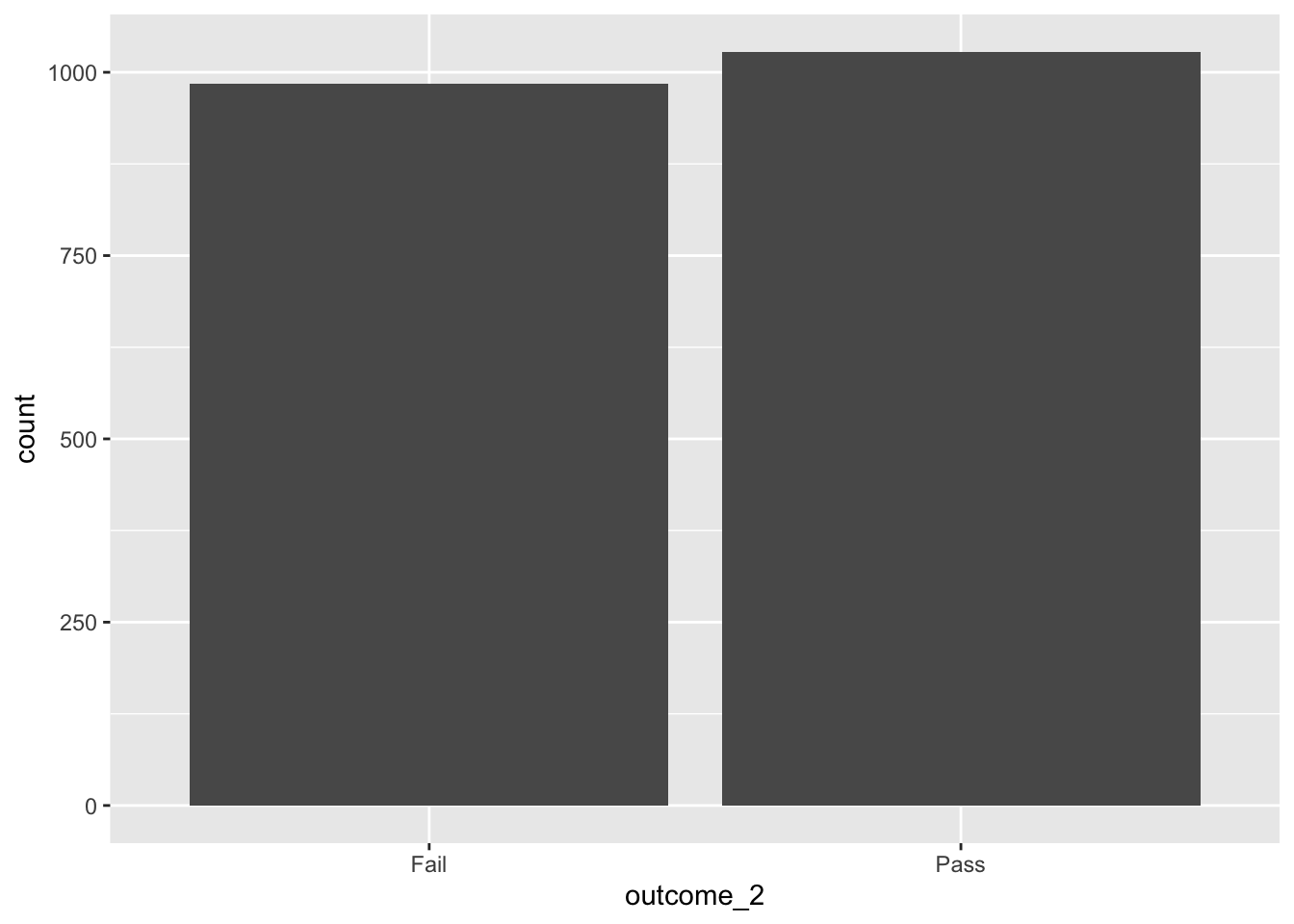``df %>% count(outcome_2)``
``````## # A tibble: 2 x 2
##   outcome_2     n
##   <chr>     <int>
## 1 Fail        985
## 2 Pass       1028``````

We note that the `outcome_2` response is more or less evenly split between the `Fail` (“positive”) and `Pass` (“negative”) classes.

``````df %>% ggplot(mapping = aes(xA)) +
geom_bar()``````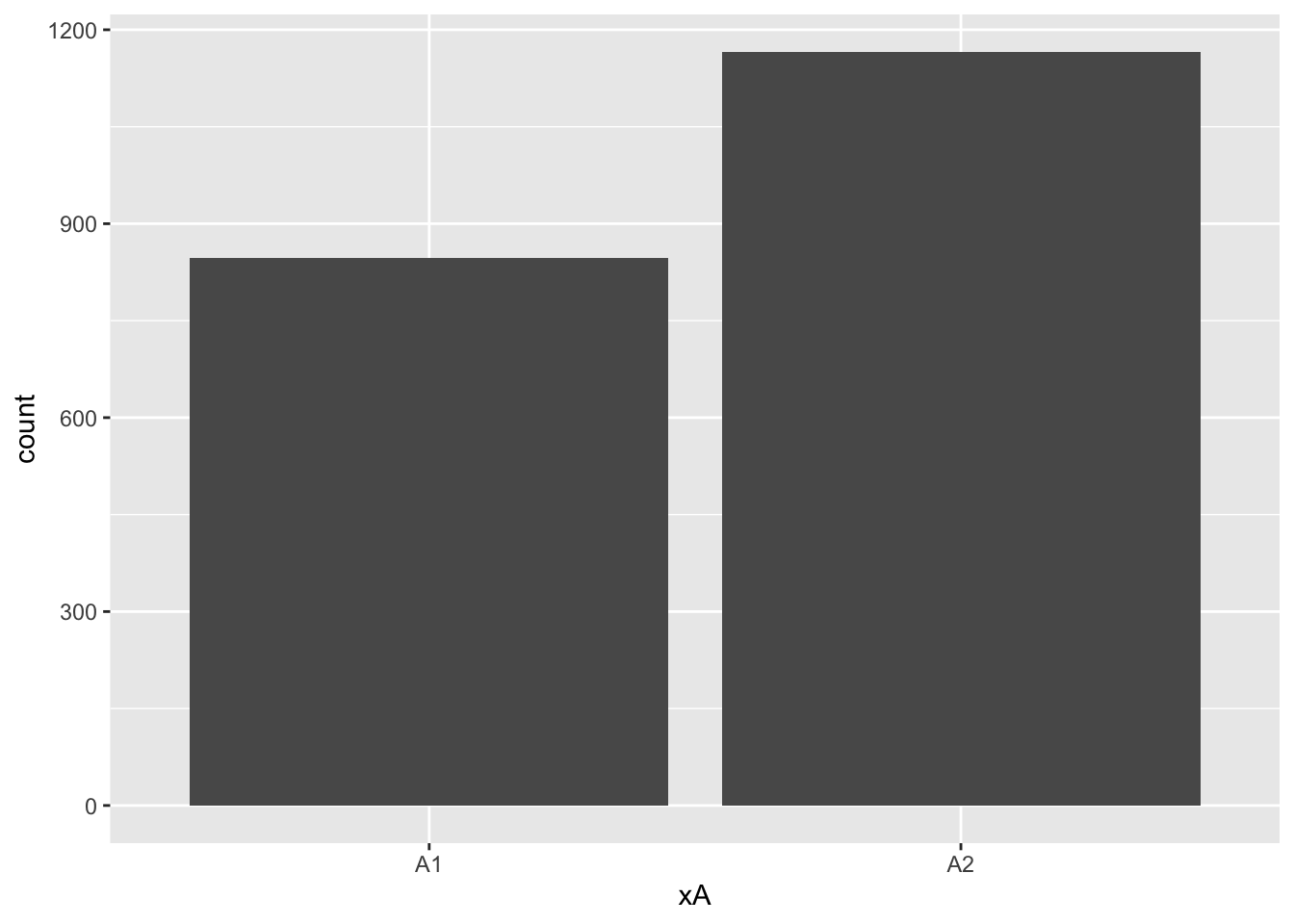``df %>% count(xA)``
``````## # A tibble: 2 x 2
##   xA        n
##   <chr> <int>
## 1 A1      847
## 2 A2     1166``````

`xA` is a categorical variable of two classes, `A1` and `A2`, with a higher proportion of `A2`.

``````df %>% ggplot(mapping = aes(xB)) +
geom_bar()``````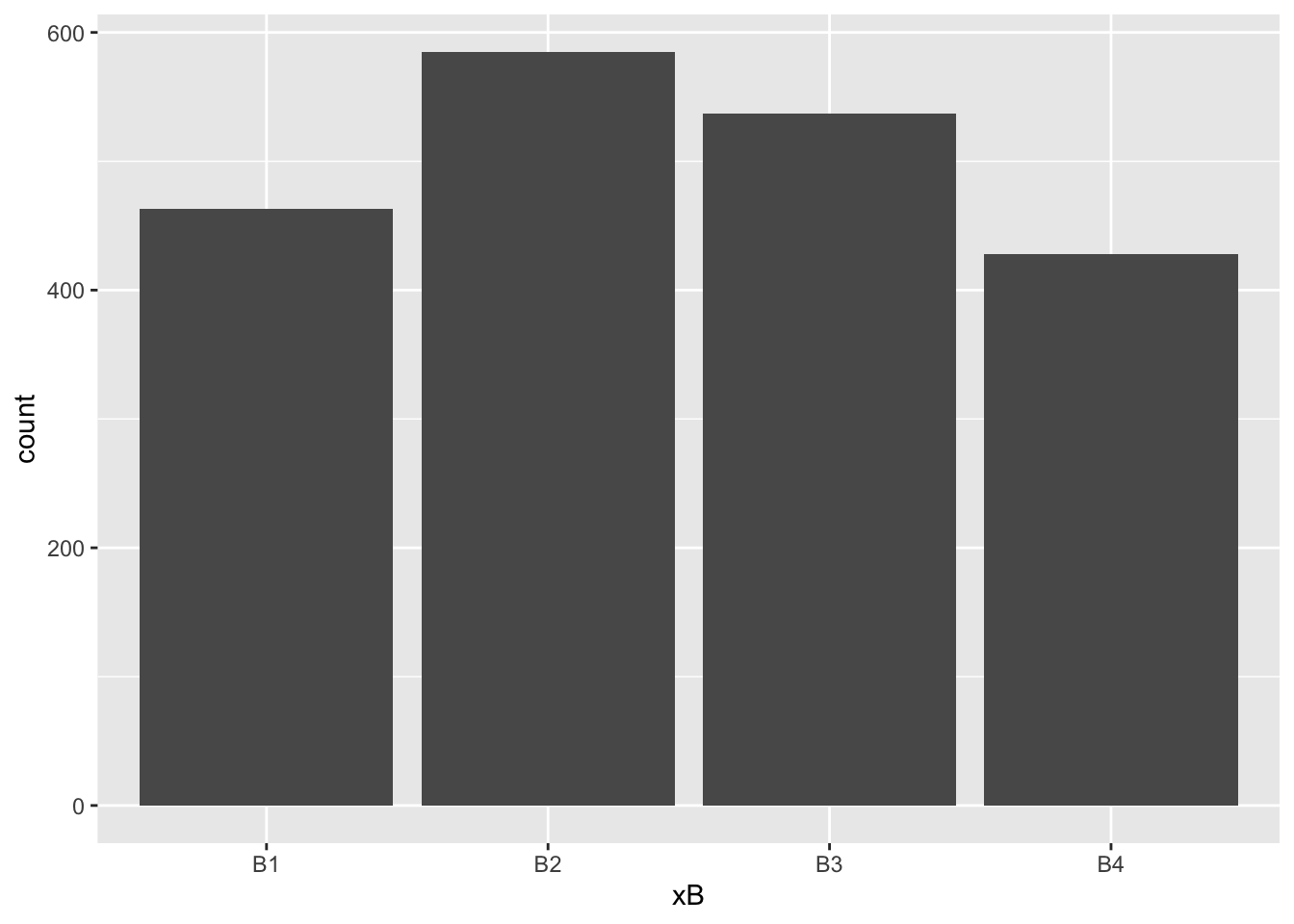``df %>% count(xB)``
``````## # A tibble: 4 x 2
##   xB        n
##   <chr> <int>
## 1 B1      463
## 2 B2      585
## 3 B3      537
## 4 B4      428``````

`xB` is a categorical variable of four classes, `B1` to `B4`, with higher proportions of `B2` and `B3`.

Visualizing distributions of variables `x01` to `x06`.

``````step_1_df %>% tibble::rowid_to_column() %>%
tidyr::gather(key = "key", value = "value", -rowid, -response_1, -xA, -xB) %>%
mutate(input_number = as.numeric(stringr::str_extract(key, "\\d+"))) %>%
ggplot(mapping = aes(x = value)) +
geom_histogram(bins = 31) +
facet_wrap(~input_number, scales = "free") +
theme_bw() +
theme(axis.text.y = element_blank())``````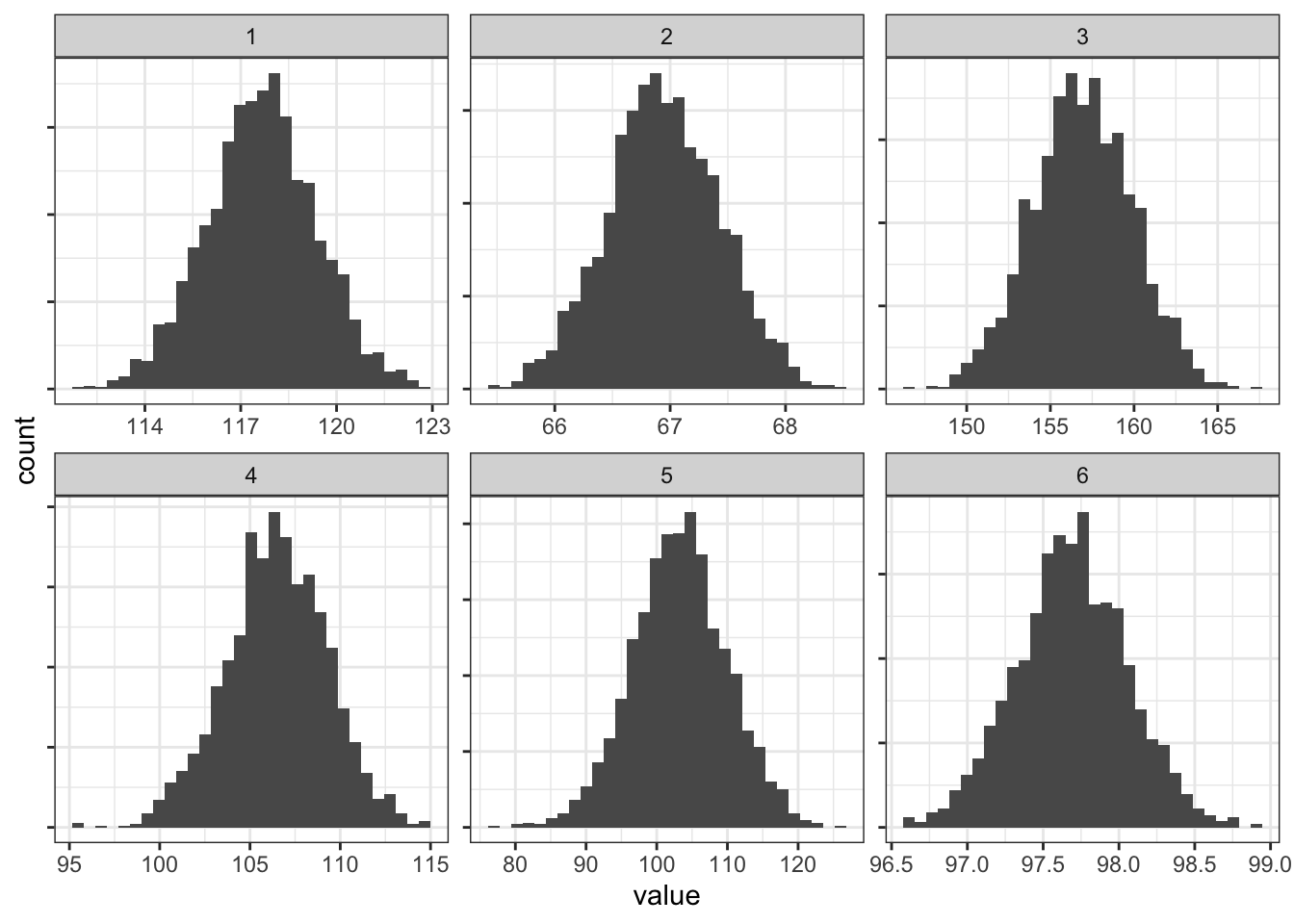All inputs model normal distributions.

``````df %>% ggplot(mapping = aes(x01)) +
geom_histogram(bins = 30)``````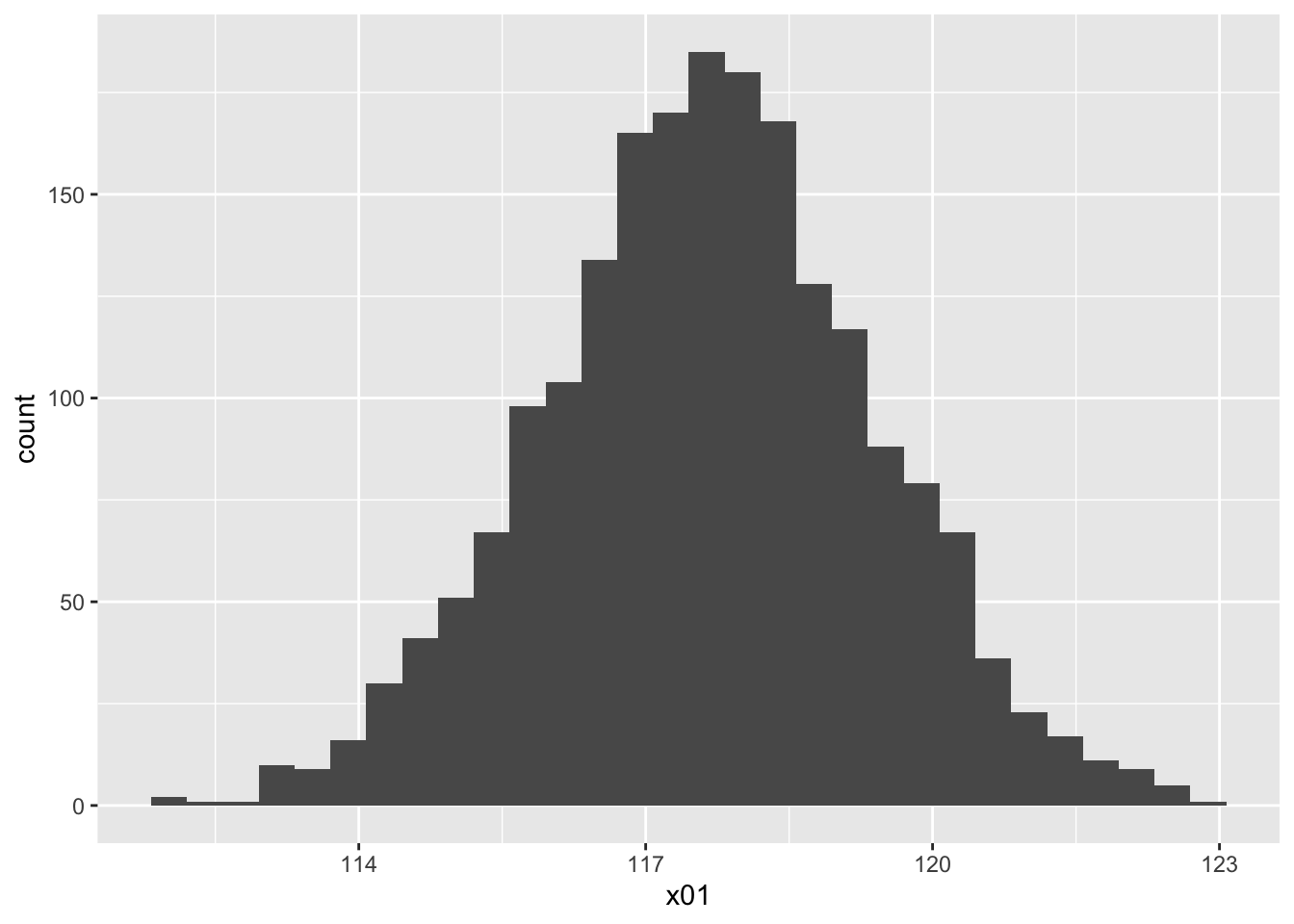``````df %>% ggplot(mapping = aes(x02)) +
geom_histogram(bins = 30, fill = 2, alpha = 0.5)``````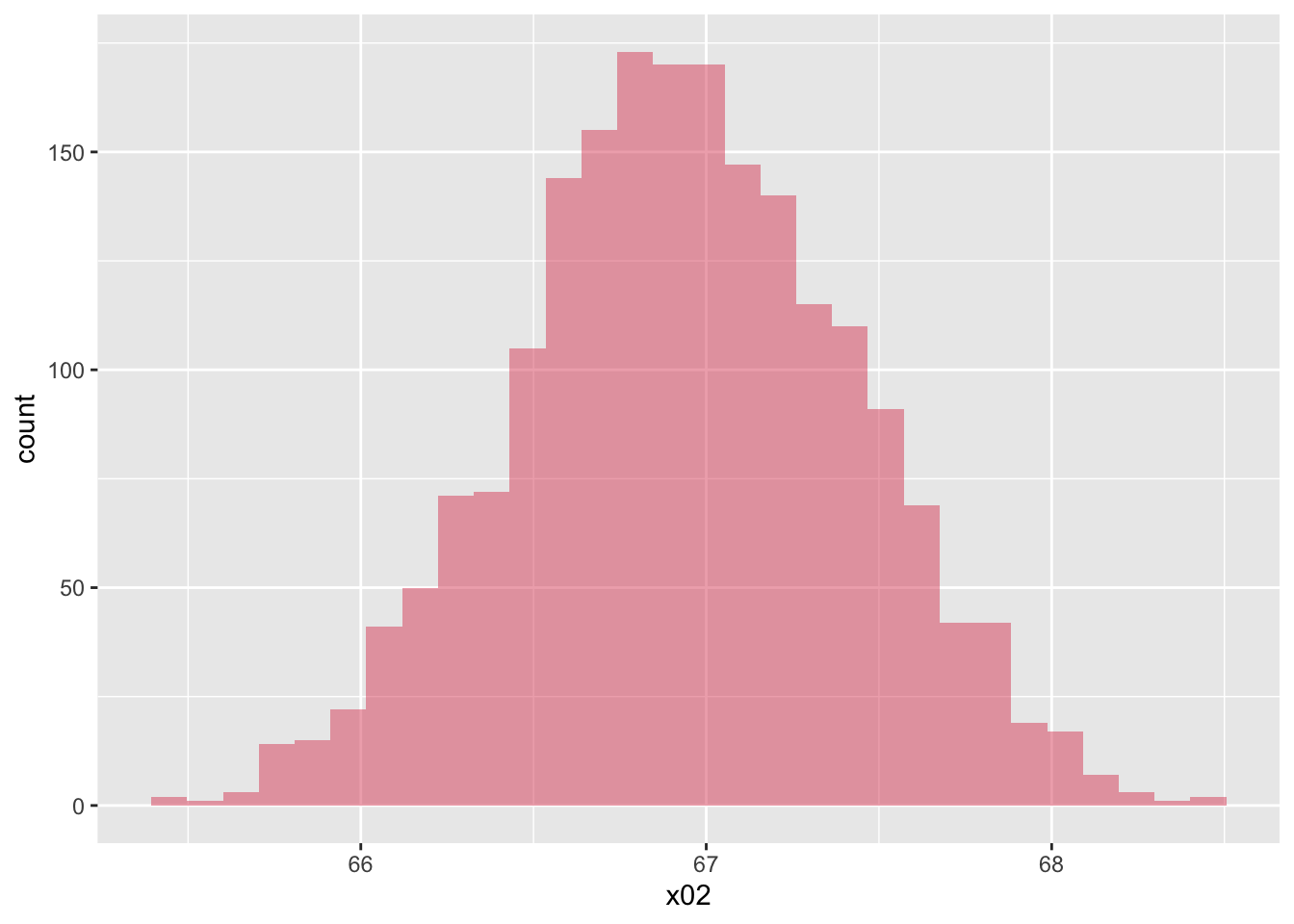``````df %>% ggplot(mapping = aes(x03)) +
geom_histogram(bins = 30, fill = 3, alpha = 0.5)``````